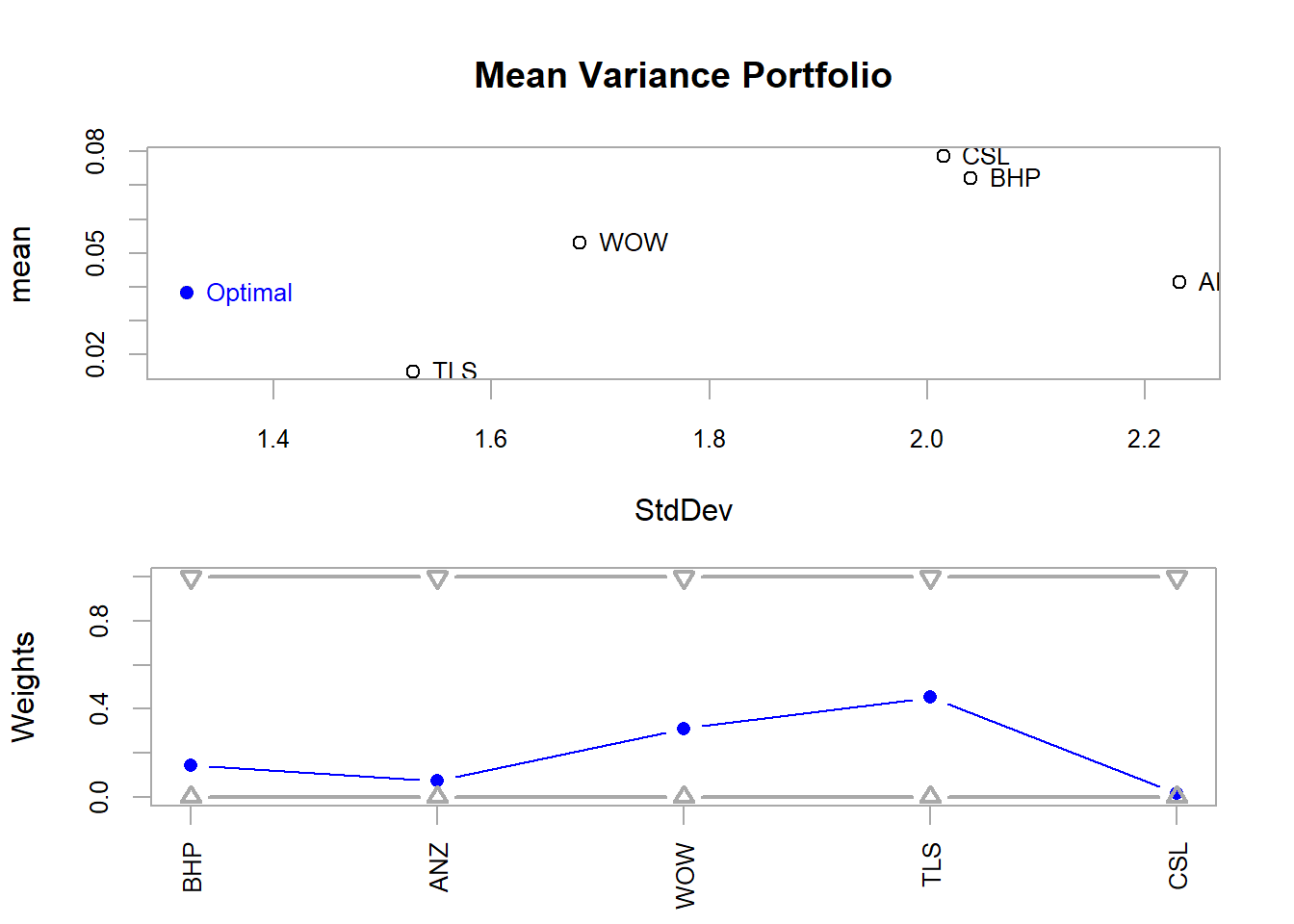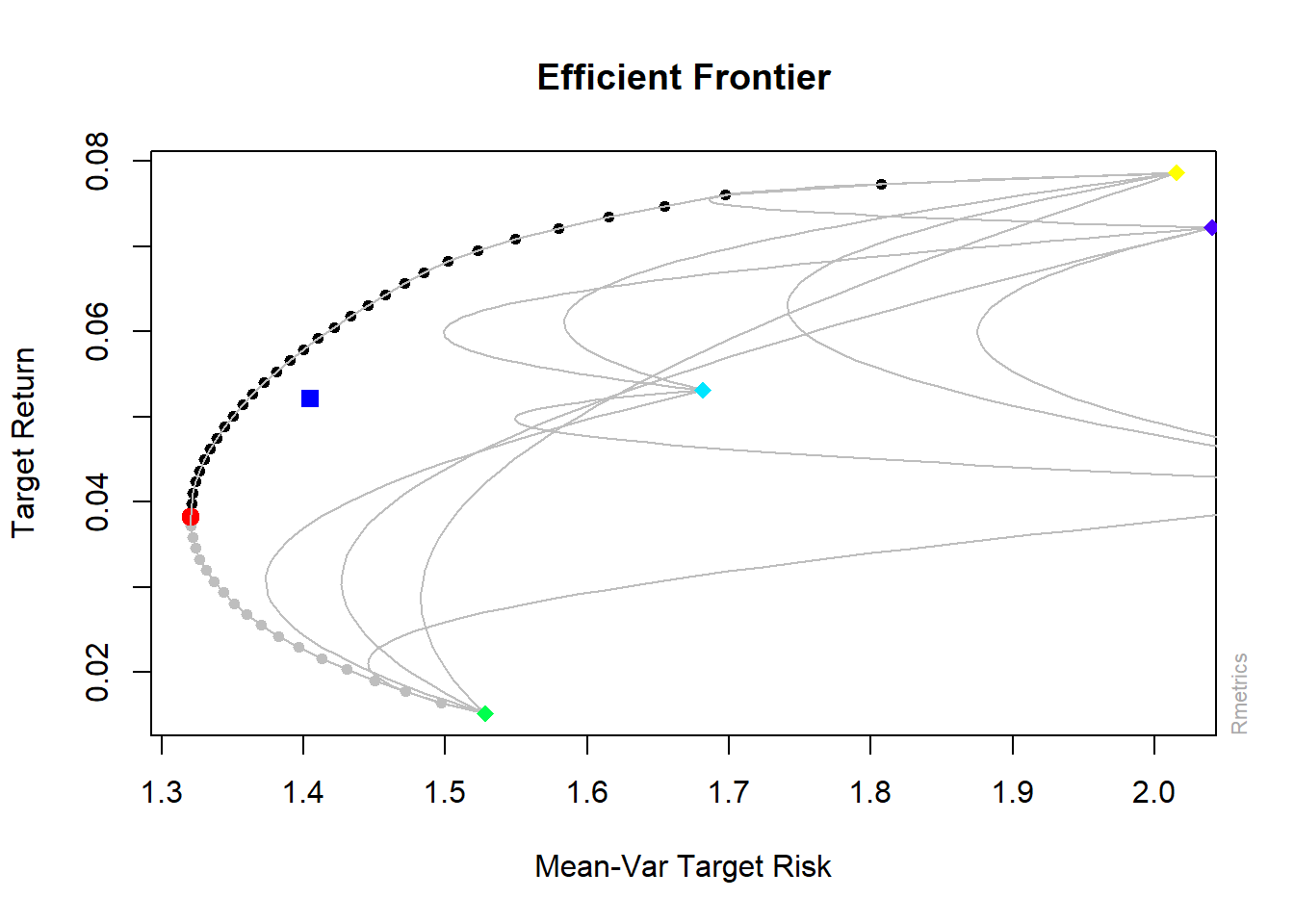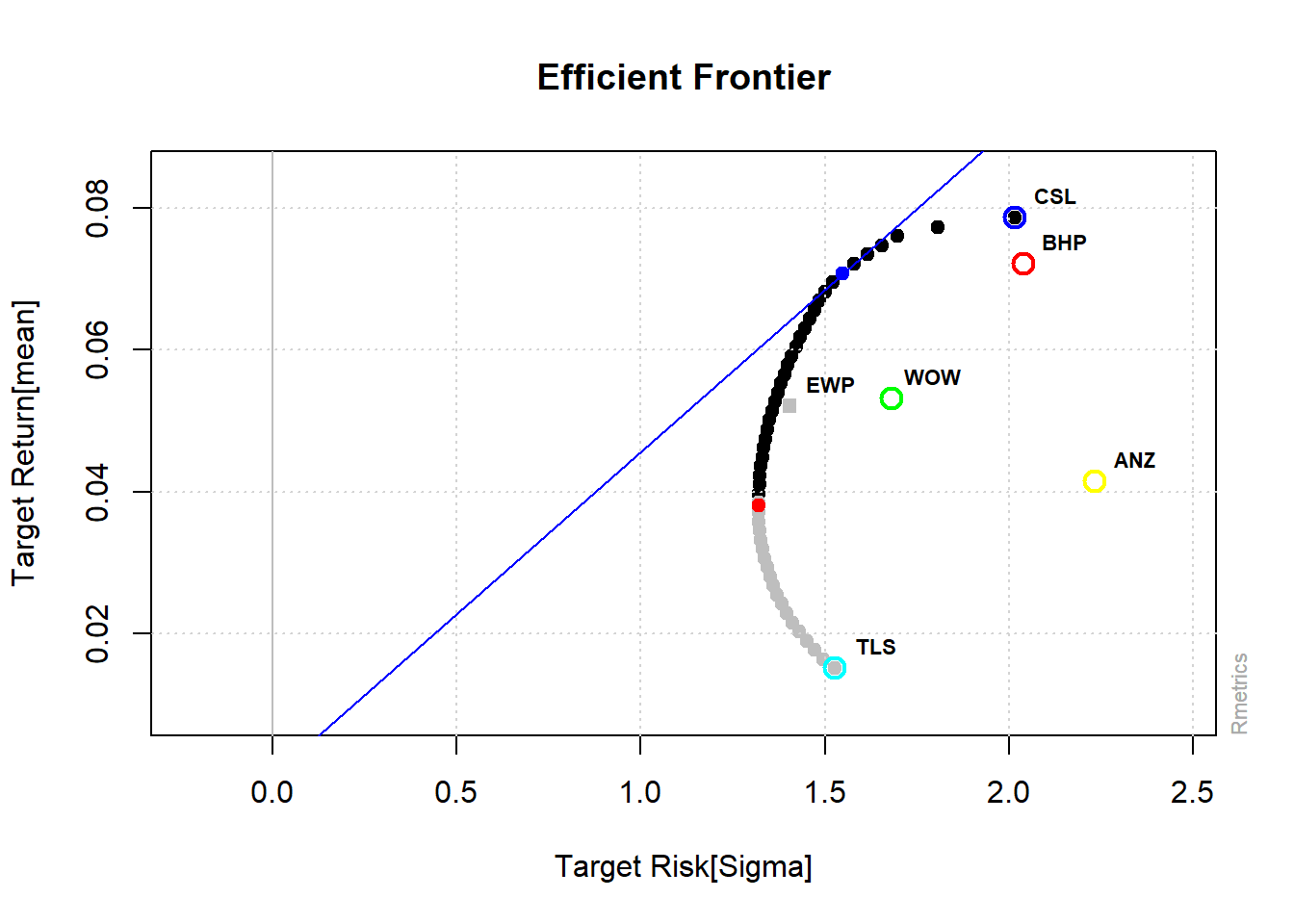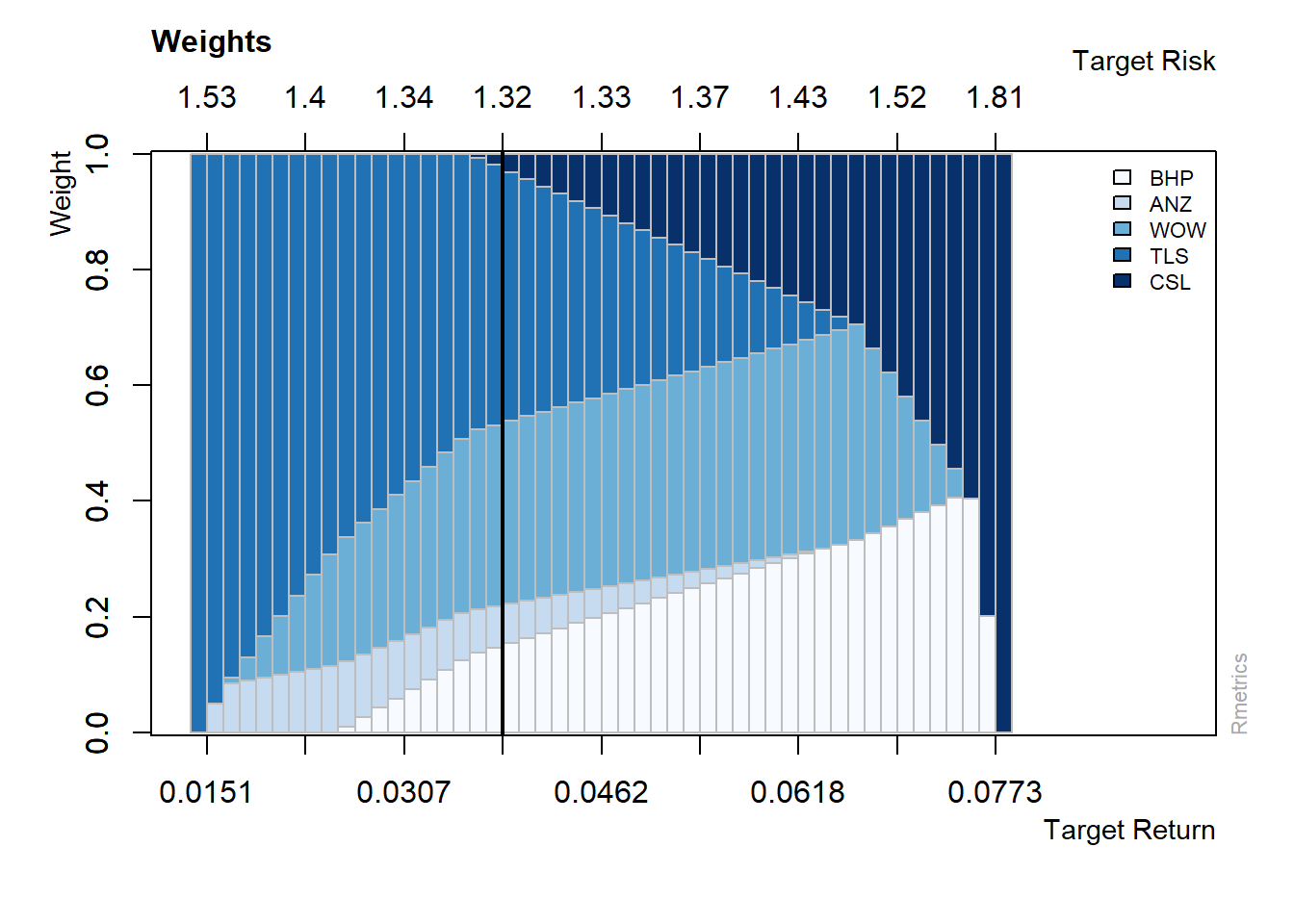## 12.6 Efficient (Minimum Variance) Portfolio using R packages

• R’s PortfolioAnalytics package provides various tools for portfolio anlaytics including minimum variance portfolio optimisation
• Create random portfolios based on risk (Std. Dev.) and reward (mean return)
• Full investment: Allocate in all the assets (minimum 0 and maximum 1 weight)
• Long only: Only buy, no short position (positive weights only)
library(PortfolioAnalytics)
# initialise with asset names uses time series data
data_p2 = zoo(ret1[, -1], order.by = as.Date(ret1\$Date))
# create specification
port = portfolio.spec(assets = c(colnames(data_p2)))
port = add.constraint(portfolio = port, type = "long_only")
port = add.constraint(portfolio = port, type = "full_investment")

# objective: manimise risk
port_rnd = add.objective(portfolio = port, type = "risk", name = "StdDev")

# objective: maximise return
port_rnd = add.objective(portfolio = port_rnd, type = "return", name = "mean")

# 1. optimise random portfolios

rand_p = optimize.portfolio(R = data_p2, portfolio = port_rnd, optimize_method = "random",
trace = TRUE, search_size = 1000)
# plot

chart.RiskReward(rand_p, risk.col = "StdDev", return.col = "mean", chart.assets = TRUE)  #also plots the equally weighted portfolioFigure 12.5: Mean-Variance Portfolios

• Optimise for minimum risk
• Minimise the level of Standard Deviation for the portfolio
port_msd = add.objective(portfolio = port, type = "risk", name = "StdDev")
minvar1 = optimize.portfolio(R = data_p2, portfolio = port_msd, optimize_method = "ROI",
trace = TRUE)
minvar1
***********************************
PortfolioAnalytics Optimization
***********************************

Call:
optimize.portfolio(R = data_p2, portfolio = port_msd, optimize_method = "ROI",
trace = TRUE)

Optimal Weights:
BHP    ANZ    WOW    TLS    CSL
0.1437 0.0735 0.3122 0.4543 0.0164

Objective Measure:
StdDev
1.32 
# plot
plot(minvar1, risk.col = "StdDev", main = "Mean Variance Portfolio", chart.assets = TRUE)Figure 12.6: Mean Variance Portfolio Risk/Return

# efficient frontier
minvar_ef = create.EfficientFrontier(R = data_p2, portfolio = port_msd,
type = "mean-StdDev")
chart.EfficientFrontier(minvar_ef, match.col = "StdDev", type = "l", tangent.line = FALSE,
chart.assets = TRUE)Figure 12.7: Efficient Frontier

library(fPortfolio)
data_p2 = as.timeSeries(data_p2)
pspec = portfolioSpec()  #initial specification

setNFrontierPoints(pspec) = 500  #random portfolios for the efficient frontier

eff_front2 = portfolioFrontier(data_p2, constraints = "LongOnly")  #strategy
plot(eff_front2, c(1, 2, 4, 5, 6))Figure 12.8: Efficient frontier plot (fPortfolio)

• Another function (allows to add different points and lines from other portfolios)
• Also plots of the weights in different portfolios
tailoredFrontierPlot(eff_front2, sharpeRatio = FALSE, risk = "Sigma")Figure 12.9: Efficient Frontier

# weights
weightsPlot(eff_front2)Figure 12.10: Weights Plot

### 12.6.1 Minimum Variance and portfolio for a given level of return

• Minimum variance
• Uses previous constraints (Long Only)
(minvar2 = minvariancePortfolio(data_p2))

Title:
MV Minimum Variance Portfolio
Estimator:         covEstimator
Optimize:          minRisk
Constraints:       LongOnly

Portfolio Weights:
BHP    ANZ    WOW    TLS    CSL
0.1437 0.0735 0.3122 0.4543 0.0164

Covariance Risk Budgets:
BHP    ANZ    WOW    TLS    CSL
0.1437 0.0735 0.3122 0.4543 0.0164

Target Returns and Risks:
mean    Cov   CVaR    VaR
0.0382 1.3204 3.2805 2.0550

Description:
Mon Oct 25 17:30:39 2021 by user: RMachine 
• Target Return
mu = mean(colMeans(data_p2))  #target return
setTargetReturn(pspec) = mu
(eff_port2 = efficientPortfolio(data_p2, pspec))

Title:
MV Efficient Portfolio
Estimator:         covEstimator
Optimize:          minRisk
Constraints:       LongOnly

Portfolio Weights:
BHP    ANZ    WOW    TLS    CSL
0.2364 0.0342 0.3425 0.2360 0.1510

Covariance Risk Budgets:
BHP    ANZ    WOW    TLS    CSL
0.2563 0.0326 0.3439 0.1994 0.1677

Target Returns and Risks:
mean    Cov   CVaR    VaR
0.0521 1.3608 3.4011 1.9698

Description:
Mon Oct 25 17:30:39 2021 by user: RMachine 

### 12.6.2 Portfolio with Box Constraints

• We can add box constraints to setup minimum weights so that we invest in each stock in the portfolio.
• This can be achieved using both packages.
• Here we demonstrate using the fPortfolio package. See help(box_constraints) for PortfolioAnalytics package
pspec2 = portfolioSpec()

setNFrontierPoints(pspec) = 500
boxconstraints = c("minW[1:5]=0.1", "maxW[1:5]=1")  #for minimum asset weights and maximum asset weights
eff_front3 = portfolioFrontier(data_p2, spec = pspec2, constraints = boxconstraints)
eff_front3

Title:
MV Portfolio Frontier
Estimator:         covEstimator
Optimize:          minRisk
Constraints:       minW maxW
Portfolio Points:  5 of 24

Portfolio Weights:
BHP    ANZ    WOW    TLS    CSL
1  0.1000 0.1000 0.1248 0.5752 0.1000
6  0.1160 0.1000 0.2713 0.4127 0.1000
12 0.1900 0.1000 0.3253 0.2611 0.1236
18 0.2323 0.1000 0.3382 0.1292 0.2003
24 0.2558 0.1000 0.1000 0.1000 0.4442

Covariance Risk Budgets:
BHP    ANZ    WOW    TLS    CSL
1  0.0922 0.0983 0.1022 0.6022 0.1052
6  0.1136 0.1040 0.2662 0.4063 0.1099
12 0.2038 0.1069 0.3268 0.2270 0.1354
18 0.2495 0.1038 0.3270 0.0972 0.2225
24 0.2495 0.0917 0.0711 0.0669 0.5209

Target Returns and Risks:
mean    Cov   CVaR    VaR
1  0.0346 1.3525 3.2604 2.0459
6  0.0410 1.3280 3.3011 2.0827
12 0.0488 1.3482 3.3697 1.9793
18 0.0566 1.3977 3.4827 2.0566
24 0.0644 1.5223 3.7541 2.2943

Description:
Mon Oct 25 17:30:39 2021 by user: RMachine 
plot(eff_front2, c(1, 2, 4, 5, 6))Figure 12.11: Frontier Plot (box constraints)

(minvar3 = minvariancePortfolio(data = data_p2, spec = pspec2, constraints = boxconstraints))

Title:
MV Minimum Variance Portfolio
Estimator:         covEstimator
Optimize:          minRisk
Constraints:       minW maxW

Portfolio Weights:
BHP    ANZ    WOW    TLS    CSL
0.1179 0.1000 0.2728 0.4093 0.1000

Covariance Risk Budgets:
BHP    ANZ    WOW    TLS    CSL
0.1158 0.1041 0.2680 0.4021 0.1099

Target Returns and Risks:
mean    Cov   CVaR    VaR
0.0412 1.3280 3.3025 2.0801

Description:
Mon Oct 25 17:30:41 2021 by user: RMachine